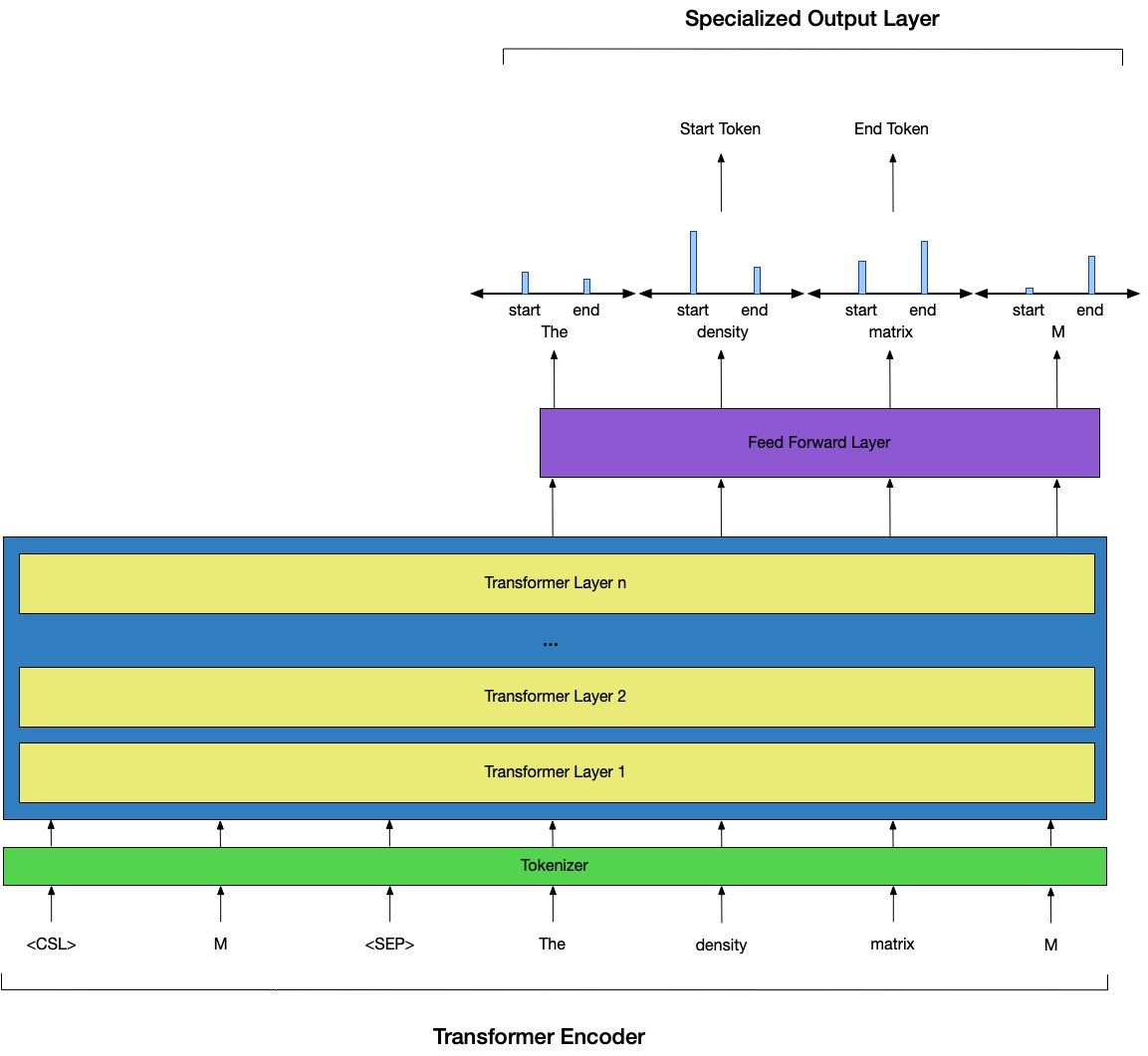# Mathematical token definition extraction (aka identifier definition extraction)

## Embedding of Mathermatical Expresssions

#### Problem

Mathematical formulae are a foundational component of information in all scientific and mathematical papers. Parsing meaning from these expressions by extracting textual descriptors of their variable tokens is a unique challenge that requires semantic and grammatical knowledge. In this research, we developed a new manually-labeled dataset of mathematical objects, the contexts in which they are defined, and their textual definitions. With this dataset, we evaluate the accuracy of a number of modern neural-network models on two definition extraction tasks. While this is not a solved task, modern language models such as BERT perform very well ($\sim$90%). Both the dataset and neural-network models (implemented in PyTorch jupyter-notebooks) are available online to help aide future researchers in this space.

## People

###### Emma Hamel
Alumni Research Assistant